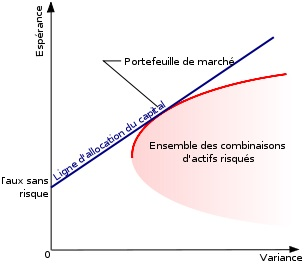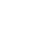# Model of Evaluation of assets financial or Capital Asset Pricing ModelThe model of evaluation of financial assets (CAPM), translation of the English Capital Asset Pricing Model (CAPM) provides an estimate of the rate of return expected by the market for a financial asset on the basis of its systematic risk.

# The DEDAF is the best-known form of modern portfolio theory

The DEDAF is the most well-known form of the modern portfolio theory which is a financial theory developed in 1952 by Harry Markowitz. It exposes how rational investors use diversification to optimize their portfolio, and what should be the price of an asset given its risk compared with the average market risk. This theory uses the concepts of efficient frontier, coefficient beta, right of market capital and right of securities market. Its most accomplished formalization is the financial asset pricing model or CAPM.

# Assumptions

To operate the CAPM assumes the following theoretical assumptions as true:

1. There are no transaction costs (no commission, and no margin bid – ask).
2. An investor can buy or sell short any action without impact on the share price.
3. There are no taxes (in particular, it is the same for capital gains and dividends).
4. Investors do not like risk.
5. Investors have the same time horizon.
6. All investors a Markowitz portfolio because they consider each action what aspects mean-variance.
7. Investors control risk by diversification.
8. All assets, including human capital, can be bought and sold freely on the market.
9. Investors can lend or borrow money to risk-free rate.

Can graphically represent the assessment of financial assets (CAPM) model. Take first right of capital market (capital market line (CML) in English). An investor considers the expected performance [E(R)]and risk (variability represented by the standard deviation of the portfolio,). For a given risk, he prefers the portfolio which has the highest yield. Among all possible portfolios, Markowitz proposes to calculate those who have the highest yield for a given risk. We then get the border of Markowitz A – B. Efficient portfolios lie on this curve, which is the same for all investors (same expectations of returns and risks).

For the return of the title without risk (for example, the USA Treasury zero coupon bonds).

The right of capital market share of and is tangent to the line A – B.

An investor can choose any portfolio on the right between and M.

All investors must have the market portfolio M in more or less large proportions. For example, the H portfolio includes in equal share title without risk and portfolio M.• Rx the profitability of the risky asset
• Β the sensitivity of the action (or sector) expected by the market.
• RF the profitability of a risk-free assets.
• RM the average profitability of the market (as measured by an index).

The 'beta' of the assets is a measure of its sensitivity to market, i.e. its systematic risk. It determines the level of performance required for an active market. If the expected performance is lower (higher), investors will sell (buy) the assets until correction of its profitability decrease (increase) of its price.

Can translate clear: profitability of l´actif = profitability risk + risk premium

(risk premium calculated by average profitability of market profitability safely all multiplied by the sensitivity of the sector)

# Graphical representation: a sail

Remember your lessons, there is a right of type y = ax + b year our case (the blue line). This supposed right represented the most efficient portfolio. C´EST to say having the highest yield for a given risk. Note that all existing assets are in pink. Only a combination of these assets because the line red and blue connect (tangent). The model of evaluation of financial assets (CAPM) or Capital Asset Pricing Model (CAPM) is represented by a sail.Markowitz also introduced the concept of efficient frontier. Each asset has a couple risk/return it is possible to represent graphically (in pink). For each performance, there is a portfolio that minimizes risk. Conversely, for each level of risk, can be found a portfolio maximizing the expected yield. All of these portfolios is called efficient frontier (in red). Here is a graphical representation. Only the red line interested investors, because c´est the line maximizing this qu´ils can get by combining portfolios, but only the blue line is the ideal portfolio. The blue line is a combination of the ideal portfolio maximum in red + l´actif without risk or credit. It n´existe qu´un ideal portfolio, it cuts the blue line and the Red (tangent).

Investors can also invest in an asset to the negligible risk and delivering a certain performance (for example, a government bond). By combining the asset without risk in a portfolio of risky assets, they can get any couple hope-variance on a right side starting from the risk-free rate and passing through the space of risky portfolios (blue line). The higher these lines only, so-called "capital allocation line", describes couples hope-variance efficient, all other less rewarding risk.

The point of tangency between the capital allocation line and the border of efficiency of risky portfolios therefore describes the only optimal combination of risky assets. At the level of the point of tangency, the optimal portfolio is composed 100% of risky assets.

All points on the line in blue ideal capital allocation represent a combination of this market and risk-free assets portfolio. Points lower than the market portfolio variance are obtained by investing partly in risk-free assets. Which means you are investing the market + portfolio of risk-free

At the level of the point of tangency, the optimal portfolio is composed 100% of risky assets.

Higher variance points describe the case where the investor gets into debt to buy the market portfolio (leverage effect).

Wholesale more diversify you your portfolio more you can get closer this famous market portfolio, because in theory the market portfolio contains all possible risky assets. To have a good portfolio theory should mix this famous portfolio and a risk-free assets.

# Risk or why it is necessary to diversify:

The specific risks of each s´annulent action when l´on diversified (all actions banks for example), a portfolio diversified so a specific risk of each significant action… The market portfolio does not specific risk.# Reviews:

There are many criticisms on the model of evaluation of financial assets (CAPM) or Capital Asset Pricing Model (CAPM).

• Correlations between different assets are not constant, they evolve over time
• The distribution curve is Gaussian and does not take into account rare events such as financial crises or the market crashes.
• Investors are not rational (behavioral finance)
• Few investors have a portfolio of Markovitz (investors non-rationalite)

• The expected profitability of the market in the CAPM formula is impossible to calculate. It uses, so an approximation that distorts the result

• The size of the companies is not taken into account. However low capitalization securities often have returns greater than large cap companies.

The CAPM assumes the following theoretical assumptions that are all open to criticism:

1. There are no transaction costs (no commission, and no margin bid – ask). However, in reality there are transaction costs and margin calls.
2. An investor can buy or sell short any action without impact on the share price. It is possible that this has an influence
3. There are no taxes (in particular, it is the same for capital gains and dividends). Now there.
4. Investors do not like risk. All do not behave with the same risk aversion, so not necessarily linear risk is premium but is more a curve.
5. Investors have the same time horizon. With the baby boomers and the boomer grandpas there is an imbalance.
6. All investors a Markowitz portfolio because they consider each action what aspects mean-variance. However, this is not the case.
7. Investors control risk by diversification. Still need qu´ils know the theory.
8. All assets, including human capital, can be bought and sold freely on the market. Gold often this is not the case.
9. Investors can lend or borrow money to risk-free rate. But no Bank lends unlimited. Harry Markowitz, in an article in the Financial Analysts Journal 2005, noted that if one modifies the assumption number 9 of the CAPM, then the conclusions of its model are changed drastically.
10. There are several Betas for a value, each making account of a macroeconomic factor sensitivity (principle of ' Arbitrage Pricing Theory). But the fundamental reasoning still stands: risk securities premiums depend on systematic risks borne by all

In addition it should be noted that at the time where Markowitz published his first work, another theory was beginning to take shape. It's that efficient markets who wants that at any time all available information is incorporated into stock prices. As a result, courses on financial markets vary at the discretion of the arrival of new information which by nature are unpredictable. No investor (except if it has privileged information) can therefore implement strategies to systematically, and level of risk given, to achieve better results than those that would be obtained by choices made randomly.

# This qu´il should remember:

Diversify more you your portfolio more it is efficient and less you risk specific d´une action.

This tool describes the relationship between the risk of a financial asset and profitability expected of this asset.

The general idea which is based by the CAPM is as follows: investors are compensated by the time value of money and risk. The time value of money is represented by the risk-free rate, which is usually at the rate of the lowest, but the least risky investment, type booklet has.

The risk is represented by the Beta, the historical relationship between the volatility of the asset and the market.## Veux-tu  commencer à construire ton patrimoine ?

Profites pour commencer à t'enrichir et exploites le système grâce à ce premier placement jusqu'à 10%
Je veux moi aussi gagner
* vous souscrivez à la newsletter richesse et finance et vous serez redirigé vers l'opportunité d'investissement du moment (lendix)## Recevez nos petits secrets pour devenir riche.

Profitez-en pour recevoir gratuitement par mails tout ce que vous avez besoin:
-les bons plans, les informations exclusives, les meilleurs conseils...
J'en profite